﻿ 基于极限平衡法和强度折减法的某滑坡体稳定性分析

# 基于极限平衡法和强度折减法的某滑坡体稳定性分析Stability Analysis of a Landslide Based on Limit Equilibrium Method and Strength Reduction Method

Abstract: The occurrence of landslide causes serious property loss and casualties. In order to ensure the safe construction of slope engineering and accurately analyze the stability of slope, the stability of landslide must be carefully analyzed. In this paper, the limit equilibrium method and the strength reduction method are used to calculate the safety factor of the slope, and the stability of the land-slide body is compared and analyzed. The calculation model of landslide mass is established by using Lizheng software and FLAC3D as calculation tools. The safety factor is calculated by simplified Janbu method and strength reduction method, and its stability is evaluated according to the code for investigation of landslide prevention engineering (gbt32864-2016). Then FLAC3D is used to calculate the stability coefficient by strength reduction method to verify the reliability of the safety coefficient. The stability of a landslide mass in Tongling is studied. The results show that the calculation and analysis results of limit equilibrium method and strength in reduction method are consistent, and the overall stability of the landslide mass is good.

1. 引言

2. 滑坡地质特征

2.1. 滑坡概况

2.2. 滑坡形成机制

3. 滑坡稳定性对比分析

3.1. 理正软件极限平衡法计算安全系数

1、极限平衡法的基本原理

2、简化Janbu法

Janbu法针对实际工程中碰到的非圆弧滑动面的问题而提出的，属于极限平衡法的一种。简化Janbu法在公式推导过程忽略条间竖向剪切力 ，计算公式如下，

${F}_{S}=\frac{{\sum }_{i=1}^{n}\left[\left({c}_{i}{b}_{i}+{W}_{i}\mathrm{tan}{\phi }_{i}\right)\frac{1}{\mathrm{cos}{\alpha }_{i}{m}_{\theta i}}\right]}{{\sum }_{i=1}^{n}{W}_{i}\mathrm{tan}{\alpha }_{i}}$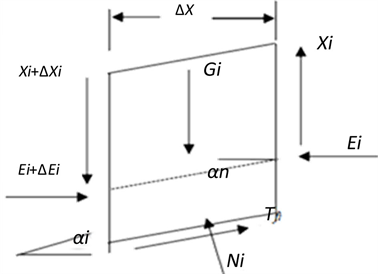Figure 1. Janbu schematic diagram of force of general bar division method

3、计算剖面和计算参数的确定

①滑坡岩土体参数取值Table 1. Value of natural severity and saturation severity of landslide rock and soil mass

②滑带土抗剪强度(C, Φ)Table 2. Value of shear strength of sliding belt soil

4、稳定性分析计算结果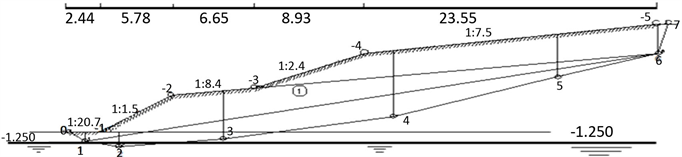Figure 2. Schematic diagram of Janbu calculation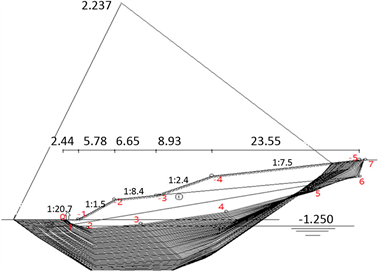Figure 3. Calculation results of JanbuTable 3. Calculation results of safety factor

3.2. FLAC3D强度折减法验算安全系数

1、强度折减法

1) 适用的地形更广，传统定性分析法只能用来判断一般地形，对于复杂场地的分析极其困难，基于历史数据进行推断复杂；

2) 可以定量描述岩土体的关系，分析变形的应力变化；模拟斜坡破坏时各阶段的应力变化；

3) 在斜坡破坏各阶段，强度折减可以捕捉滑移面的变化形态；

4) 进行斜坡抗滑设计时，支护体与坡体均可模拟；

5) 求解安全系数时，不需要做假设，也无需岩土体进行条分  。

2、强度折减法的基本原理

${c}^{trial}=\frac{c}{{F}_{trial}}$

${\phi }^{trial}=arctan\left[\frac{tan\phi }{{F}_{trial}}\right]$

3、斜坡失稳的判定

1) 利用数值确定

2) 位移骤变确定

3) 塑性区贯通确定

4、模型建立及参数的选取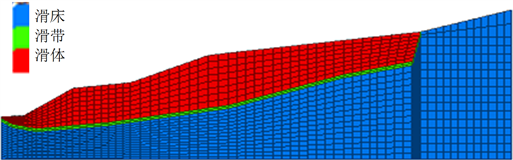Figure 4. Landslide model diagram

5、数值计算结果及分析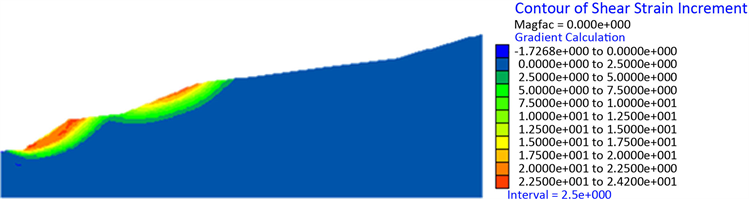Figure 5. Shear strain increment diagram

4. 结论

1) 在自然状态下，该滑坡体处于一个稳定的状态，在没有外力作用下发生滑坡的可能性不大；

2) 在暴雨状态下滑坡仍处于稳定状态，发生滑坡的可能性不大。

3) 由数值模拟方法得出的安全系数比Janbu法计算得到的安全系数略高一些，存在差异的原因在于两种方法都受到人为因素和计算模型建立简略的影响。但依据规范，得出的结论是一致的。

4) 本文采用的简化Janbu法计算简单并避免了计算不收敛，因而在边坡稳定性研究中得到了广泛应用，但该法仅在条分较密时能够满足计算。而强度折减法考虑材料间相互作用，适用条件更广。两者结合分析，结果更准确。

 章广成. 水位变化对滑坡稳定性的影响研究[D]: [硕士学位论文]. 武汉: 中国地质大学, 2005.

 Duncan, J.M. (1996) State of the Art: Limit Equilibrium and Finite-Element Analysis of Slopes. Journal of Geotechnical Engineering, 22, 577-596.
https://doi.org/10.1061/(ASCE)0733-9410(1996)122:7(577)

 Hungr, O. (1987) An Extension of Bishop’s Simplified Method of Slope Stability Analysis to Three-Dimensions. Geotechnique, 37, 113-117.
https://doi.org/10.1680/geot.1987.37.1.113

 Hungr, O., Salgado, F.M. and Byrne, P.M. (1989) Evaluation of a Three-Dimensional Method of Slope Stability Analysis. Canadian Geotechnical Journal, 26, 679-686.
https://doi.org/10.1139/t89-079

 Jing, L. (2003) A Review of Techniques Advances and Outstanding Issues in Numerical Modeling for Rock Mechanics and Rock Engineering. International Journal of Rock Mechanics and Mining Sciences, 40, 283-353.
https://doi.org/10.1016/S1365-1609(03)00013-3

 赵尚毅, 郑颖人, 时卫民, 等. 用有限元强度折减法求边坡稳定安全系数[J]. 岩士工程学报, 2002, 24(3): 343-346.

 刘金龙, 栾茂田, 赵少飞, 等. 关于强度折减有限元方法中边坡失稳判据的讨论[J]. 岩土力学, 2005, 26(8): 1345-1348.

 红保, 曹平, 林杭, 赵延林. 采用边坡稳定性强度折减法分析弹性应变能突变判据[J]. 中南大学学报(自然科学版), 2009, 40(4): 1054-1058.

 孙聪, 李春光, 郑宏, 等. 基于软化特性的三维边坡强度折减有限元分析[J]. 岩土力学, 2014, 35(SI): 407-413.

 年廷凯, 张克利, 刘红帅, 等. 基于强度折减法的三维边坡稳定性与破坏机制[J]. 吉林大学学报(地球科学版), 2013, 43(1): 178-185.

 宁宇, 徐卫亚, 郑文棠. 应用离散元强度折减对复杂边坡进行稳定性分析[J]. 岩土力学, 2007, 28(SI): 569-574.

 李志刚, 曹磊. 严格Janbu法不收敛原因及其改进的探讨[J]. 岩土力学, 2008, 29(4): 1053-1056.

 蔡志远, 马石城, 蔡志坚. 基于C语言程序的简布法边坡稳定分析[J]. 长沙大学学报, 2012, 26(5): 20-22+25.

 许冬丽, 於汝山. 三峡库区新集镇典型高切边坡破坏机理研究[J]. 路基工程, 2010(1): 42-44.

 徐文杰, 胡瑞林, 岳中琦, 谭儒蛟, 李仁江, 曾如意. 虎跳峡龙蟠右岸边坡稳定性的数值模拟[J]. 岩土工程学报, 2006, 28(11): 1996-2004.

 蒋鑫, 邱延峻, 凌建明. 强度折减法软件在评价边坡稳定性上之比较[J]. 地下空间与工程学报, 2008, 4(2): 302-309.

 黄鸿, 王遐莽. 高速公路边坡稳定性FLAC3D分析案例[J]. 公路交通科技(应用技术版), 2014, 10(1): 83-85.

 梁艳, 李同春. 边坡稳定分析有限元强度折减法失稳判据探讨[J]. 三峡大学学报(自然科学版), 2012, 34(2): 28-31.

Top